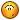BrainDen.com - Brain Teasers

## Question

Each of three circles passes through the centers of the other two.

The area interior to all three is quite close to, but different from, one quarter of the area A of a circle.

Can you determine, through inference, construction or calculation, whether it's smaller or larger than A/4?

Caveat:

Arrgh. Forgot that I posted this problem before;* where it was solved by calculation.

I'll bend the Den's rules a bit and leave it up, but modified.

Please provide a purely geometrical solution.

*A benefit of advancing age is the ability to hide one's own Easter eggs.## Recommended Posts

• 0Less than 1/4.

The ratio could be calculated as

M = [3(/pi/6 R^2 -S) + S]/[(/pi) R^2]

where S = /sqrt(3) R^2 /4 is the area of the triangle

M = 1/2 - 2S/[(/pi) R^2]

= 1/2 - /sqrt(3) /(2/pi)

< 1/4

As /sqrt(3) >1.6 > 1/4 (2/pi)

##### Share on other sites
• 0Don't know how to draw but hope it's understandable:

Let the center of the circles be A, B, C respectively and denote the intersecting point of circles A and B as M, that of A and C as N.

Consider the the area of AMB and ANC, together they make 2S. MAN is a straight line and is the diameter of circle A. Move the parts of AMB and ANC which are outside of the half circle MANCB and paste it to BC, there's still a blank space in the half circle.

Thus 2S < area of the half circle.

S< 1/4 area of the circle.

##### Share on other sites
• 0

Please provide a purely geometrical solution.

Nice Easter Egg indeedCompleting the picture with four more circles:

So 1/4 of a circle is one blue + one red petal + one half blue + one half red petal

And the area in question is one blue + one red petal + one red petal.

We need to if either blue or red is the biggest. If red is biggest than Area >1/4 CircleArea, else Area <1/4 CircleArea.

Construct another circle below to get a red petal inside the blue area. So 1/4 CircleArea > Area.

##### Share on other sites
• 0I used the area of a triangle (as an equilateral triangle with each leg being r, using Pythagorean theorem I got (r^2 sqrt(3))/4 , area of the circle (A = pi * r^2) and area of an arc segment (60' angle so 1/6*A) to get 1/2A - r^2*sqrt(3), which I don't know if it is bigger or smaller than 1/4A !!! I think it isn't...

*edit: Maybe I was calculating too much and missed the point... I looked at the spoiler above this and saw pictures...

back to the drawing board

Edited by Asitaka

## Join the conversation

You can post now and register later. If you have an account, sign in now to post with your account.×   Pasted as rich text.   Paste as plain text instead

Only 75 emoji are allowed.

×   Your previous content has been restored.   Clear editor

×   You cannot paste images directly. Upload or insert images from URL.